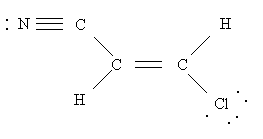# 33.39 mL of the I2 solution from above is required to titrate a sample

WK95

## Homework Statement

33.39 mL of the I2 solution from above is required to titrate a sample containing As2O3. Calculate the mass of As2O3 (197.8 g/mol) in the sample.

As2O3 + 5(H2O) + 2I2 → 2(H3AsO4) + 4HI

## The Attempt at a Solution

MolarMass_I2=254 g/mol
MolarMass_As2O3=197.8 g/mol
1 mol As2O3:2mol I2

I know I would have to get the mol of both I2 and As2O3 somehow in order to plug them into that relationship above.

I think Molarity may possibly be involved.

The problem is that while I know that there is 0.03339 L of I2, I don't know the concentration of I2 so i don't know how I can arrive at the number of mol of I2.

## Homework StatementHow many sigma bonds? pi bonds?

## The Attempt at a Solution

There are 6 sigma bonds since there are a total 6 of bonds between 6 atoms since all atoms have electrons in the s orbital.
There are 4 pi bonds between N and C, C and C, C and C, C and C. This is because each atom has electrons in orbital 2p.

Last edited:

Mentor
No way to solve the first problem without knowing concentration of iodine - but the problem states "solution from above", so most likely there were some information provided earlier.

Check what are pi bonds.

•1 person
WK95
Well... I didn't see the "solution above part". The solution of I2 has a molarity of 1.29 M. Using that, I found that the mass of As2O3 is 4.26g.

Also, pi bonds are the hybrid orbitals formed from the overlapping of 2 p orbitals.

Mentor
Also, pi bonds are the hybrid orbitals formed from the overlapping of 2 p orbitals.

Which bonds are created first - sigma, or pi? Is it possible to have a pi bond without sigma?

WK95
Which bonds are created first - sigma, or pi? Is it possible to have a pi bond without sigma?

I believe sigma bonds are created first. All ground state atoms will have s orbitals so for ground state atoms, there must always be sigma bonds right? If excited, then pi bonds can exist without sigma bonds.

Mentor
I believe sigma bonds are created first.

Good. Now, wherever there are two atoms bonded, first bond is sigma, rest - if they exist - are pi. How many sigma bonds? How many pi bonds?

WK95
Oh ok. i get it now. Thanks.